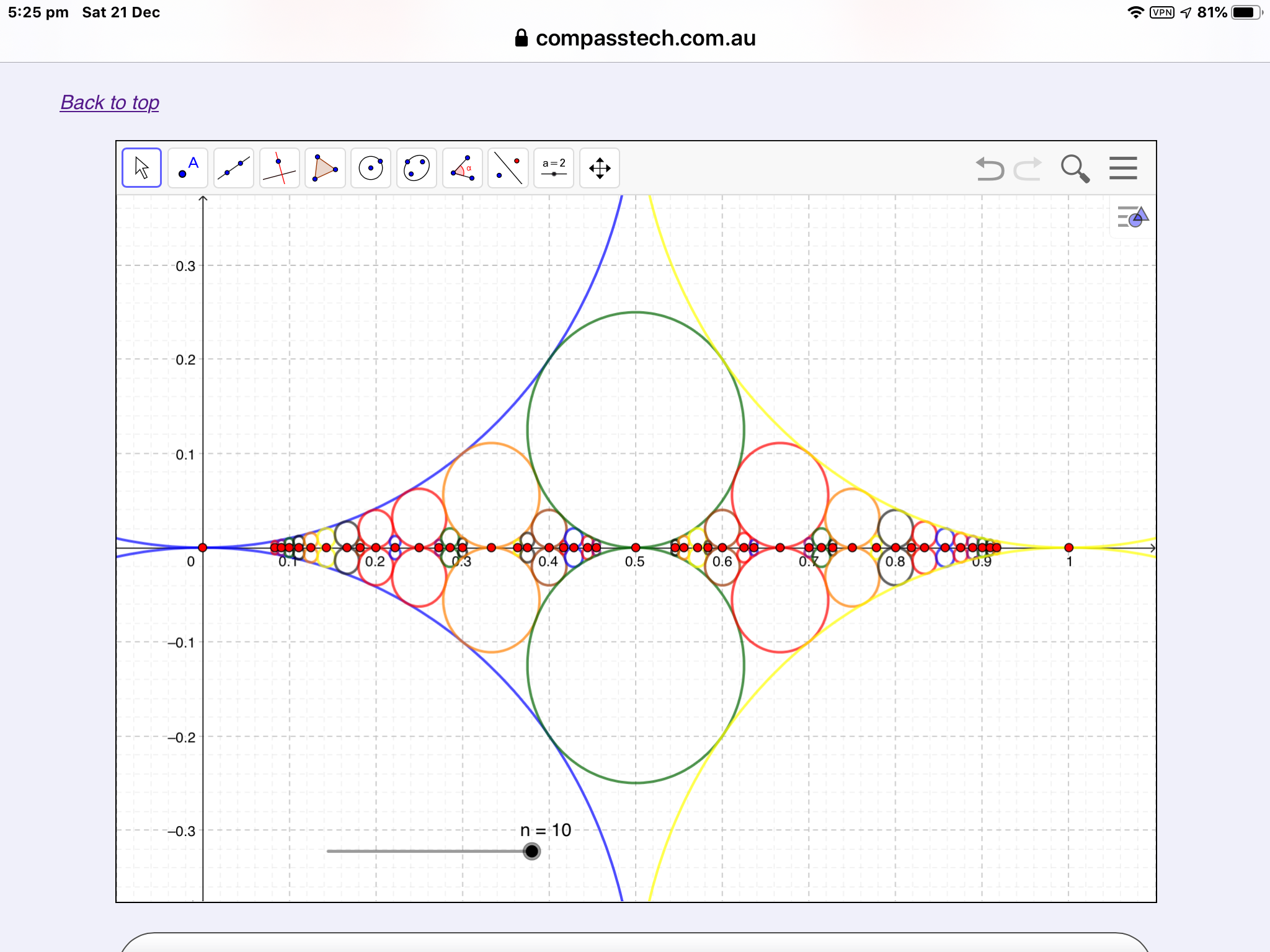©2019 Compass Learning TechnologiesLive Mathematics on the Web Check My Steps ← Ford Circles and Farey Sequences

## Ford Circles and Farey Sequences

By Dan McKinnon: Please see the blog post here.

 Suppose you wanted to make a list of all the rational numbers between 0 and 1. How would you start? One approach might be to begin with the denominators of the fractions: first, list those with denominator 1 - zero and 1. Next, add those with denominator 2: $$\frac{0}{1}, \frac{1}{2}, \frac{1}{1}$$. Add those with denominator 3 ( $$\frac{0}{1}, \frac{1}{3}, \frac{1}{2}, \frac{2}{3}, \frac{1}{1}$$) and you are on your way to the Farey Sequence! So many patterns! So much to explore... For example: suppose you wanted to know the next term of the sequence between two values, $$\frac{a}{b}$$ and $$\frac{c}{d}$$? Easy! Just add the numerators and the deniminators! $$\frac{a+c}{b+d}$$ Now plot these Farey Numbers on the number line: for each $$\frac{a}{b}$$ draw a circle of radius $$\frac{1}{2b^2}$$ and centre $$\left(\frac{a}{b},\frac{1}{2b^2}\right)$$. These are the Ford Circles that you see above! Now try plotting the numerators of a Farey Sequence against the denominators. The result (when reflected in the axes and the diagonals) is a Farey Sunburst!Keep playing. Keep exploring. Have fun!

Home Live Mathematics and STEM on the Web Check My Steps ← Ford Circles and Farey Sequences